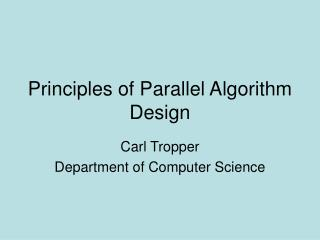DownloadDownload PresentationPrinciples of Parallel Algorithm Design

# Principles of Parallel Algorithm Design

Download Presentation## Principles of Parallel Algorithm Design

- - - - - - - - - - - - - - - - - - - - - - - - - - - E N D - - - - - - - - - - - - - - - - - - - - - - - - - - -
##### Presentation Transcript

1. Principles of Parallel Algorithm Design Carl Tropper Department of Computer Science

2. What has to be done • Identify concurrency in program • Map concurrent pieces to parallel processes • Distribute input, output and intermediate data • Manage accesses to shared data by processors • Synchronize processors as program executes

4. Matrix vector multiplication

5. Database Query • Model =civic and year=2001 and (color=green or color=white)

6. Data Dependencies

7. Another graph

8. Task talk • Task Granularity • Fine grained, coarse grained • Degree of concurrency • Average degree-average number of tasks which can run in parallel • Maximum degree • Critical path • Length-sum of the weights of the nodes on the path • Average degree of concurrency=total work/length

10. Sparse matrix multiplication • Tasks compute entries of output vector • Task i owns row i and b(i) • Task i sends non zero elements of row i to other tasks which need them

11. Sparse matrix task interaction graph

12. Process MappingGoals and illusions • Goals • Maximize concurrency by mapping independent tasks to different processors • Minimize completion time by having a process ready on the critical path when a task is ready • Map processes which communicate a lot to same processor • Illusions • Can’t do all of the above-they conflict

13. Task Decomposition • Big idea • First decompose for message passing • Then decompose for the shared memory on each node • Decomposition Techniques • Recursive • Data • Exploratory • Speculative

14. Recursive Decomposition • Good for problems which are amenable to a divide and conquer strategy • Quicksort - a natural fit

16. Sometimes we force the issue We re-cast the problem into divide and conquer paradigm

17. Data Decomposition • Idea-partitioning of data leads to tasks • Can partition • Output data • Input data • Intermediate data • Whatever………………….

18. Partitioning Output Data Each element of the output is computed independently as a function of the input

19. Other decompositions

20. Output data againFrequency of itemsets

21. Partition Input Data • Sometimes more natural thing to do • Sum of n numbers-only have one output • Divide input into groups • One task per group • Get intermediate results • Create one task to combine intermediate results

22. Top-partition inputBottom-partition input and output

23. Partitioning of Intermediate Data • Good for multi-stage algorithms • May improve concurrency over a strictly input or strictly output partition

24. Matrix Multiply Again

25. Concurrency Picture • Max concurrency of 8 vs • Max concurrency of 4 for output partition • Price is storage for D

26. Exploratory Decomposition • For search space type problems • Partition search space into small parts • Look for solution in each part

27. Search Space ProblemThe15 puzzle

28. Decomposition

29. Parallel vs serial-Is it worth it?It depends on where you find the answer

30. Speculative Decomposition • Computation gambles at a branch point in the program • Takes path before it knows result • Win big or waste

31. Speculative ExampleParallel discrete event simulation • Idea: Compute results at c,d,e before output from a is known

32. Hybrid • Sometimes better to put two ideas together

33. Hybrid • Quicksort - Recursion results in O(n) tasks, little concurrency. • First decompose, then recurse (a poem)

34. Mapping Tasks and their interactions influence choice of mapping scheme

35. Task Characteristics Task generation Static- know all tasks before algorithm executes • Data decomposition leads to static generation Dynamic-runtime • Recursive decomposition leads to dynamic generation • Quicksort

36. Task Characteristics • Task sizes • Uniform, non-uniform • Knowledge of task sizes • 15 puzzle: don’t know task sizes • Matrix multiplication: do know task sizes • Size of data associated with tasks • Big data can cause big communication

37. Task interactions • Tasks share data, synchronization information, work • Static vs dynamic • Static-know task interaction graph and when interactions happen before execution • Parallel matrix multiply • Dynamic • 15 puzzle problem

38. More interactions • Regular versus irregular • Interaction may have structure which can be used • Regular: image dithering • Irregular: sparse matrix multiplication • Access pattern for b depends on structure of A

39. Image dithering

40. Data sharing • Read only- parallel matrix multiply • Read-write • 15 puzzle • Heuristic search:estimate number of moves to solution from each state • Use priority queue to store states to be expanded • Priority queue contains shared data

41. Task interactions • One way • Read only • Two way • Producer consumer style • Read-write (15 puzzle)

42. Mapping tasks to processes Goal Reduce overhead caused by parallel execution So • Reduce communication between processes • Minimize task idling • Need to balance the load • But these goals can conflict

43. Balancing load is not always enough to avoid idling Task dependencies get in the way Processes 9-12 can’t proceed until 1-8 finish MORAL: Include task dependency information in mapping

44. Mappings can be • Static-distribute tasks before algorithm executes • Depends on task size, size of data, task interactions • NP complete for non-uniform tasks • Dynamic-distribute tasks during algorithm execution • Easier with shared memory

45. Static Mapping • Data partitioning • Results in task decomposition • Arrays, graphs common ways to represent data • Task partitioning • Task dependency graph is static • Know task sizes